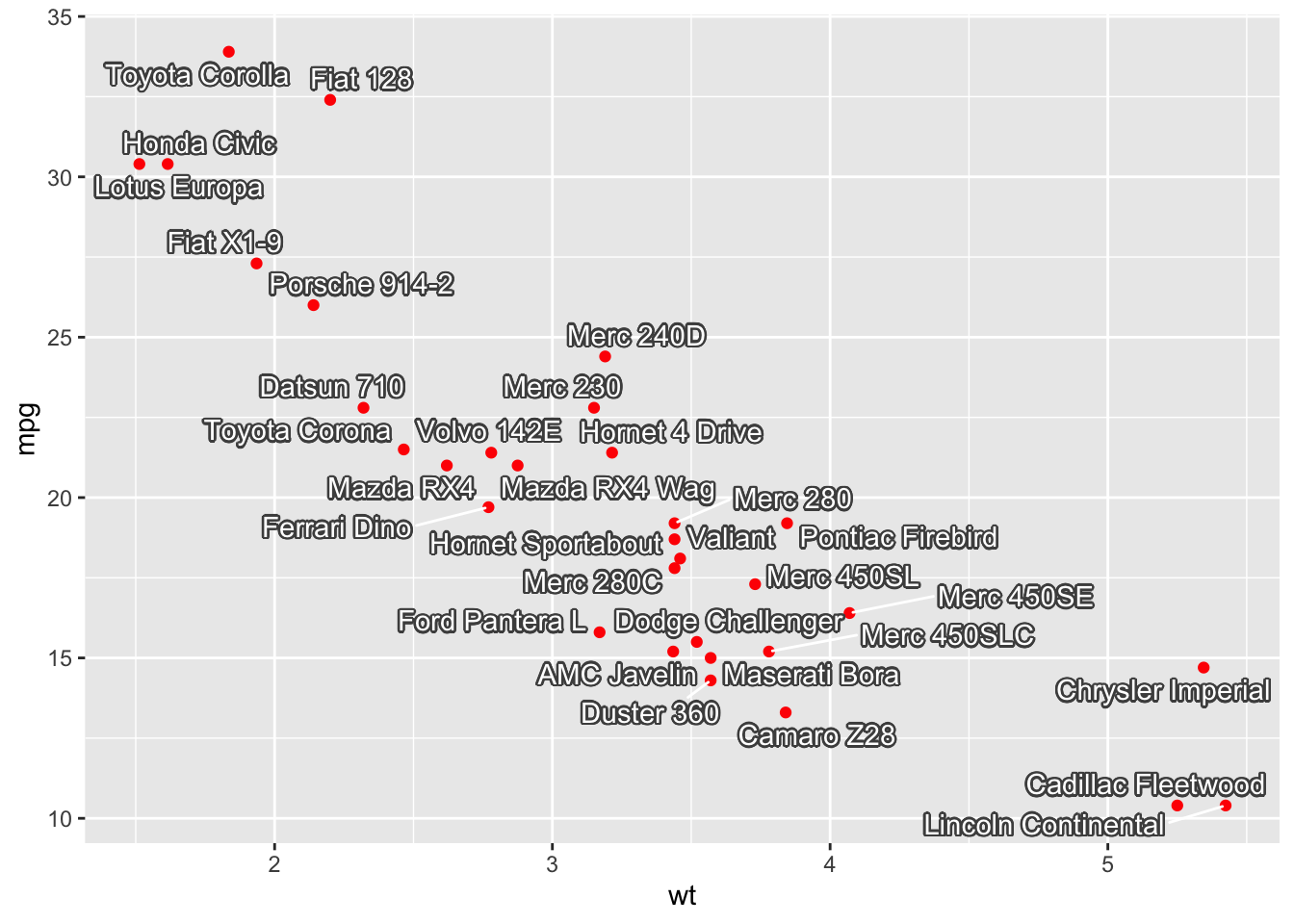# Avoid overlapping text with ggrepel

This post explains how to avoid overlapped text with automatic positioning in ggplot2 plots using the `ggrepel` package.
This post showcases the key features of `ggrepel` and provides a set of graph examples using the package.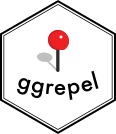{ggrepel}

# Quick start

The `ggrepel` package in R is an extension of the ggplot2 package, designed to simplify the process of avoiding overlapped texts in plots.

It offers 2 main functions: `geom_text_repel()` and `geom_label_repel()`

✍️ author → Kamil Slowikowski

📘 documentationgithub

⭐️ more than 1000 stars on github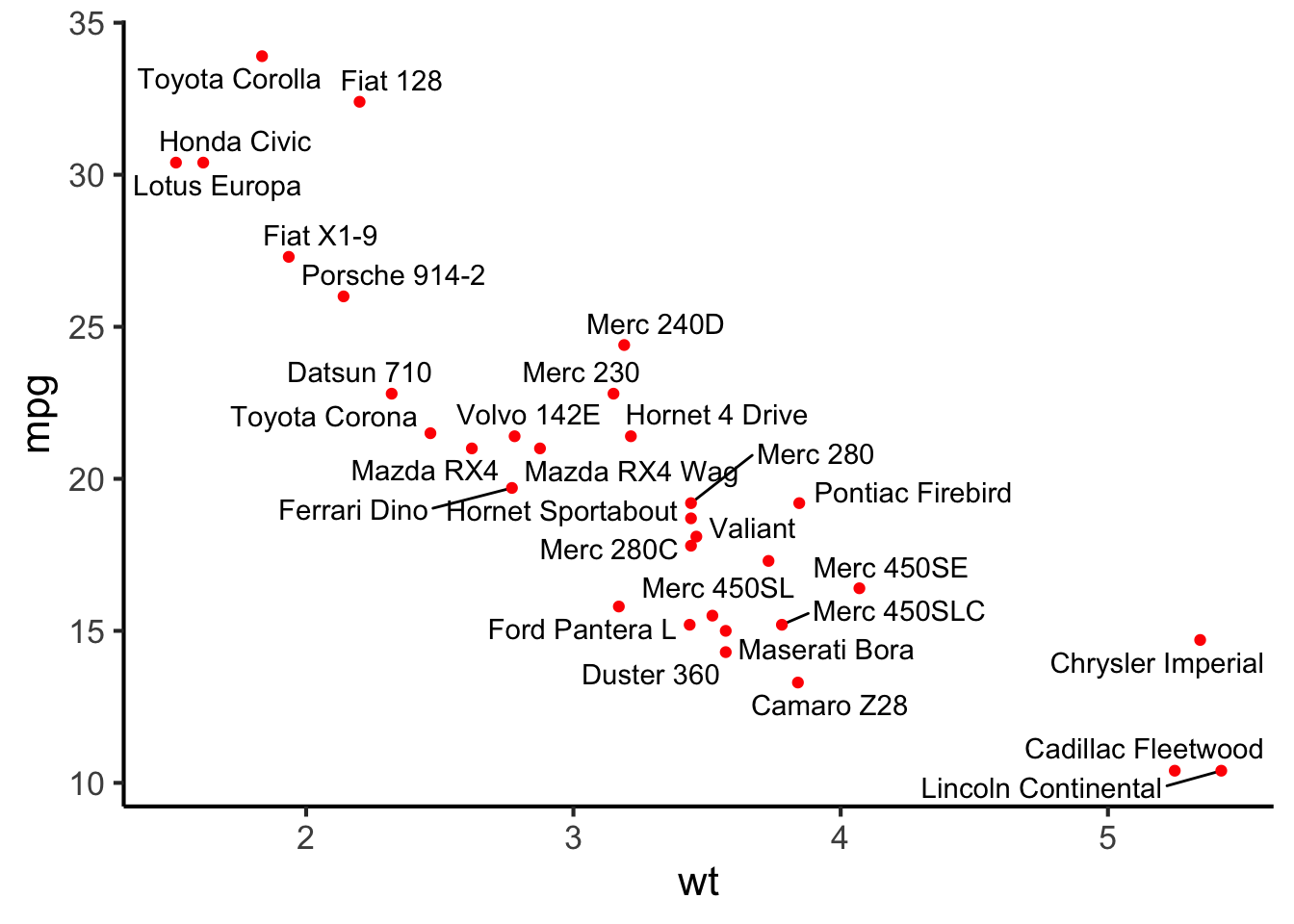# Installation

To get started with `ggrepel`, you can install it directly from CRAN using the `install.packages` function:

``install.packages("ggrepel")``

# Basic usage

The `ggrepel` package allows you to display labels using a single geom and invert the functions `geom_text_repel()` and `geom_label_repel()` without constraint:

Here’s a basic example:

``````library(ggplot2)
library(ggrepel)
data(iris)

ggplot(iris, aes(Sepal.Width, Sepal.Length, label=Species, color=Species)) +
geom_text_repel()``````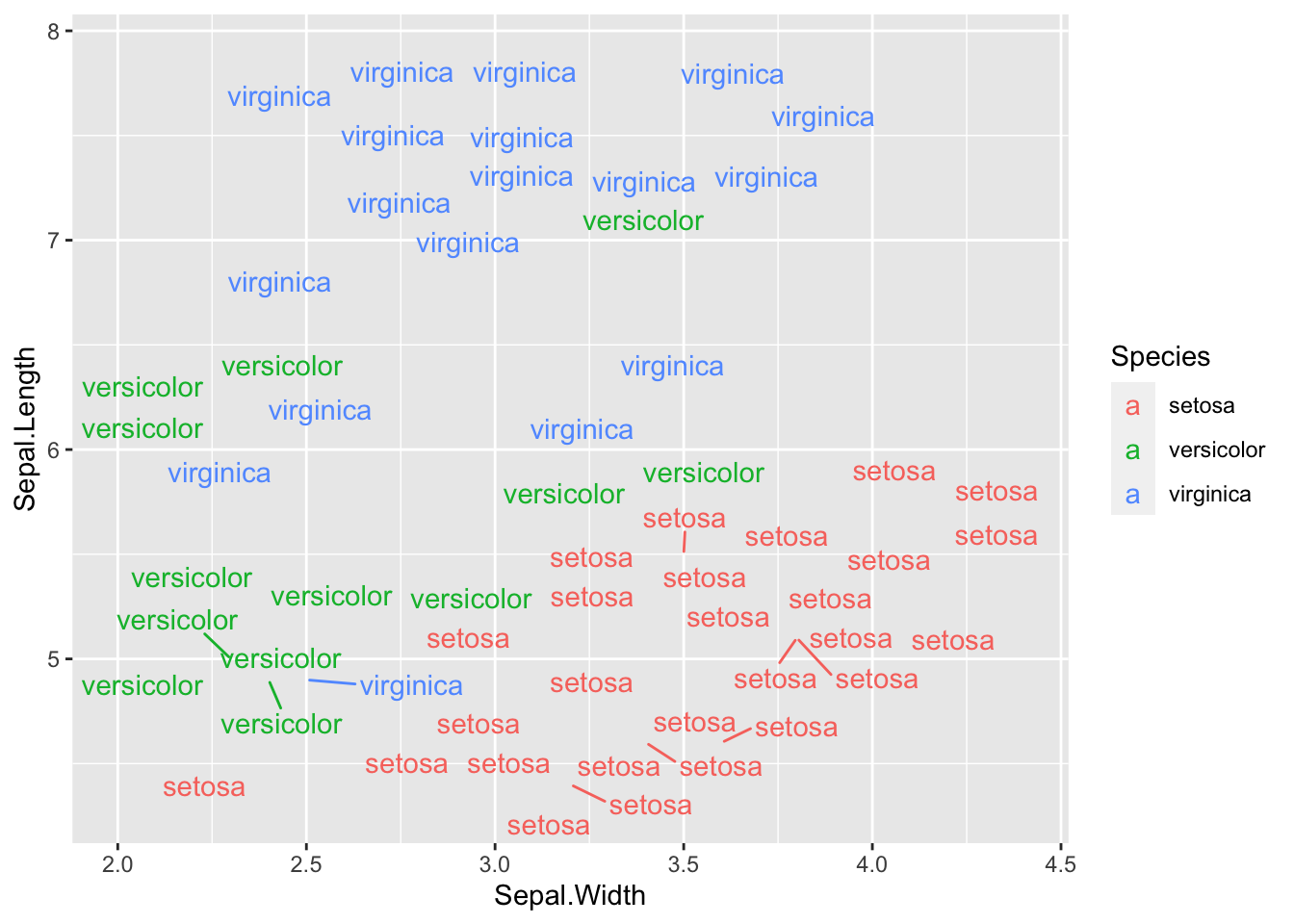# Key features

## → Combining scatter plot with labels

You can labels on individual data points with the `geom_label_repel()` function. All points will not be displayed if there is not enough room for them, but you can force it with the max.overlaps argument (check below).

Example:

``````library(ggplot2)
library(ggrepel)
data(iris)

ggplot(iris, aes(Sepal.Width, Sepal.Length, label=Species, color=Species)) +
geom_label_repel() +
geom_point() +
theme_classic(base_size = 16) +
theme(legend.position = "none") ``````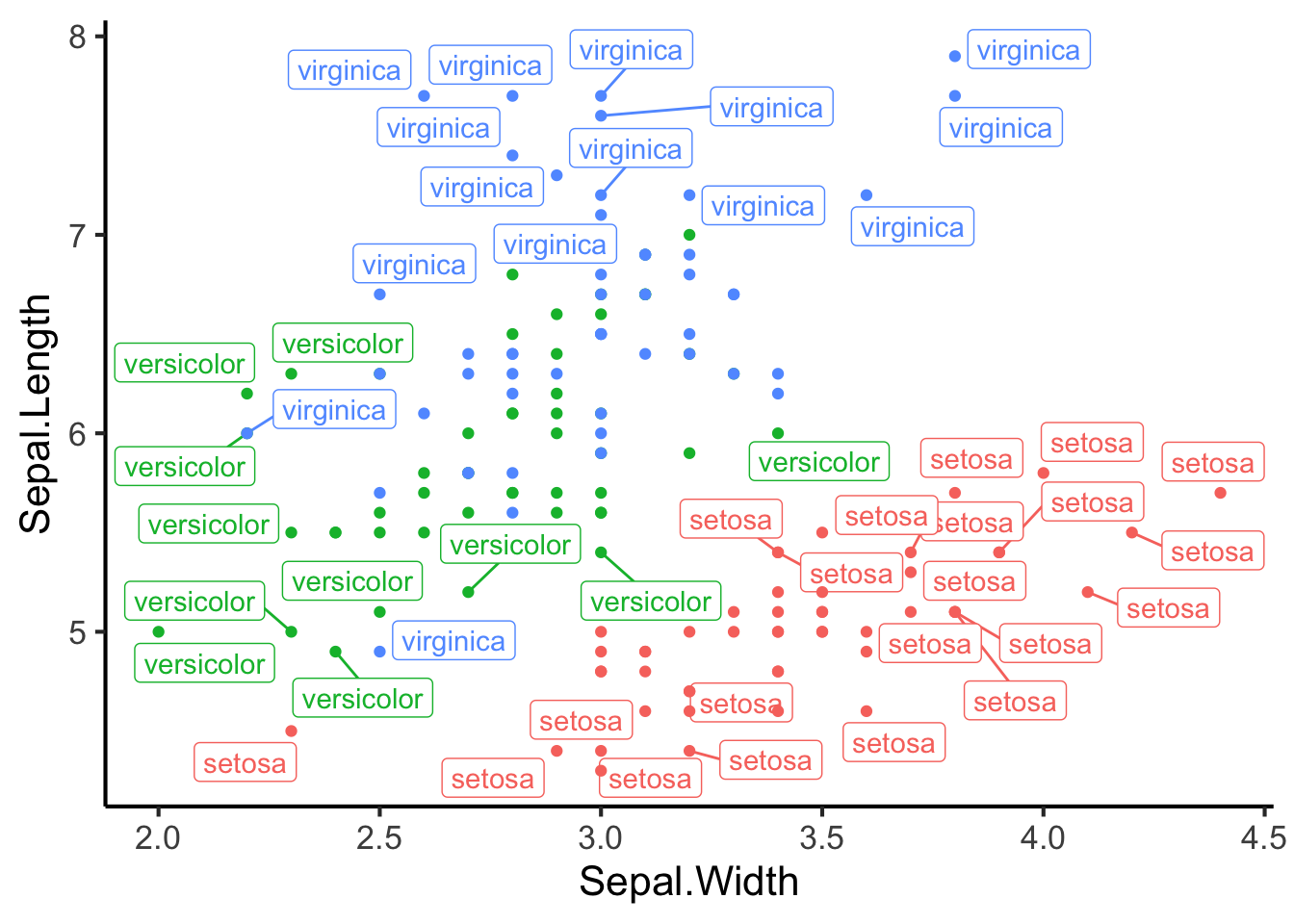## → Display only some specific labels

The easiest way to display only some labels is to create a new column on your dataframe with a non-empty value only for the observations you’re interested in.

Example:

``````# Create a new columns with the label only for row 2,3 and 14
mtcars\$car = ""
idx_to_label = c(2, 20, 14)
mtcars\$car[idx_to_label] = rownames(mtcars)[idx_to_label]

# Display the result
ggplot(mtcars, aes(wt, mpg, label = car)) +
geom_text_repel() +
geom_point(color = ifelse(mtcars\$car == "", "grey50", "red"))``````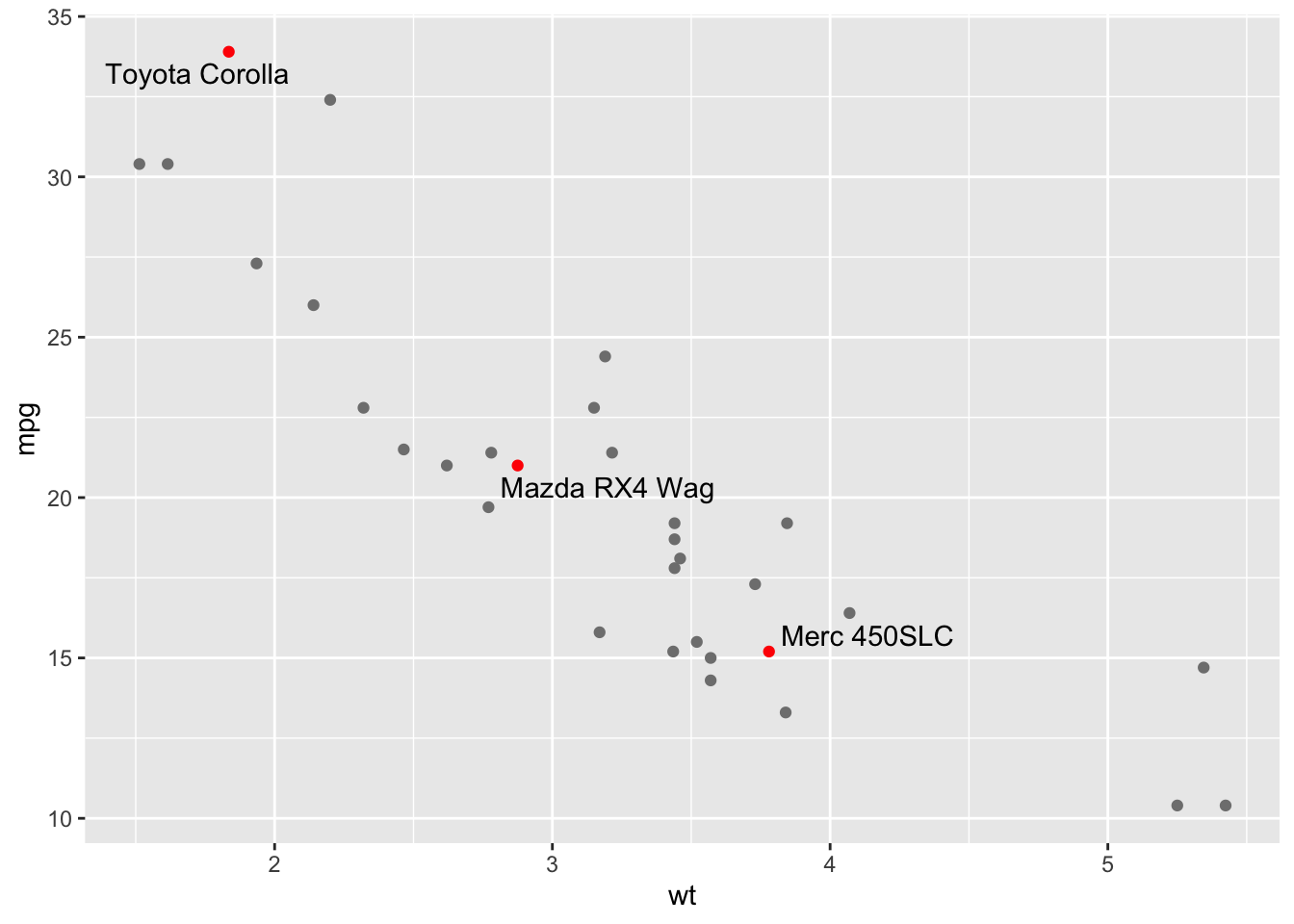## → For maximum overlapping

The `geoms` provided by `ggrepel` try do avoid as much as possible overlapping, with a maximum of 10 by default. However, you can change this value if overlapping is not a problem for you with the `max.overlaps` argument!

Example:

``````library(ggplot2)
library(ggrepel)
data(iris)

ggplot(iris, aes(Sepal.Width, Sepal.Length, label=Species, color=Species)) +
geom_text_repel(max.overlaps=nrow(iris)) + # ensure all data points are displayed
geom_point() +
theme_classic(base_size = 10) +
theme(legend.position = "none") ``````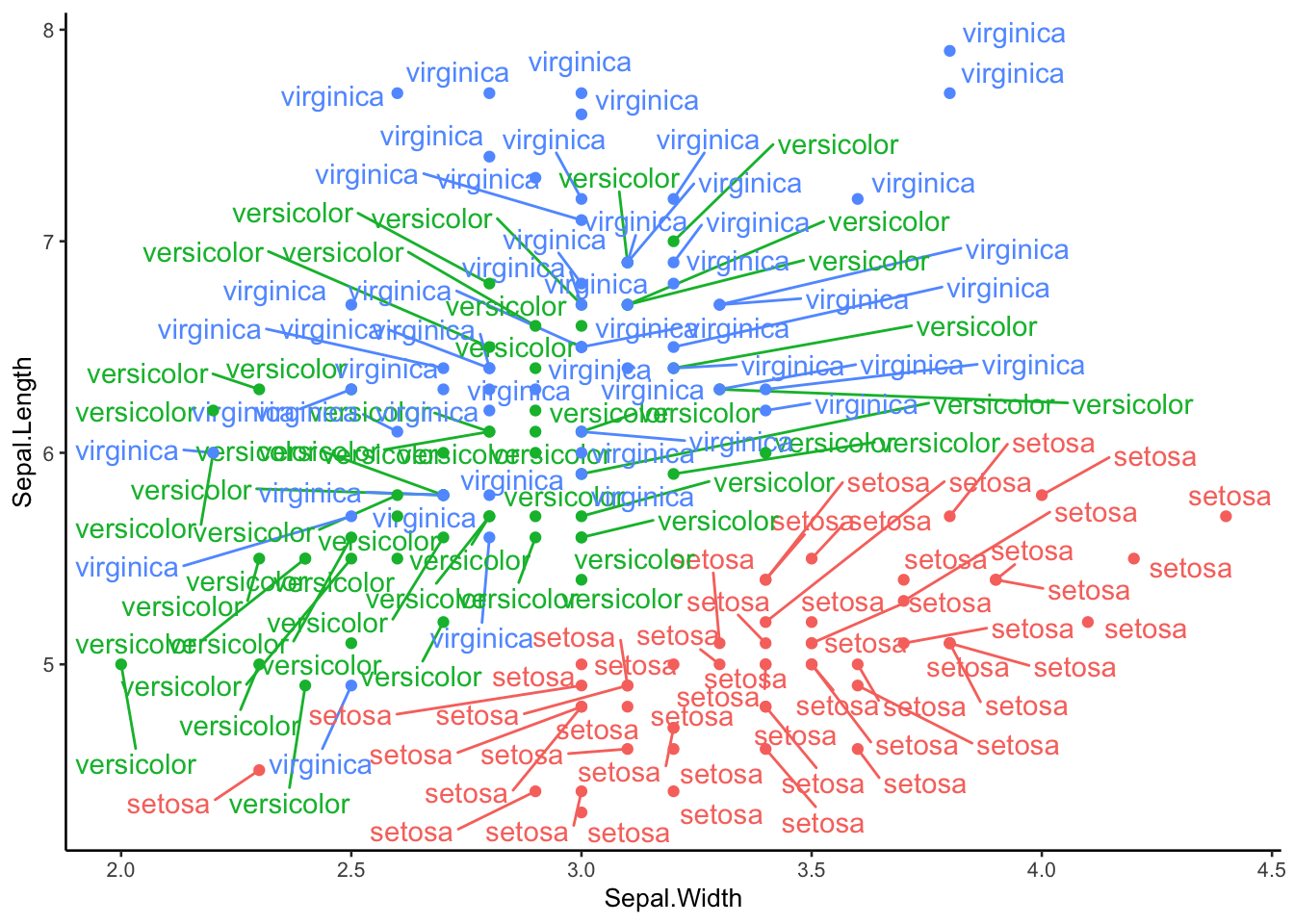## → Parallel coordinates plot

Parallel coordinates plot are easy to create with `ggrepel` combined with the `geom_segement()` function from ggplot2

Example:

``````library(hrbrthemes)
df = data.frame(x1 = 1,
y1 = rnorm(10),
x2 = 2,
y2 = rnorm(10),
lab = state.name[1:10])

ggplot(df, aes(x1, y1, xend = x2, yend = y2, label = lab, col = lab)) +
geom_segment(size = 0.5) +
guides(color = "none") + # remove legend
theme_bw() + # change background color and overall theme
geom_text_repel(nudge_x = -0.2, direction = "y", hjust = "right") +
geom_text_repel(aes(x2, y2), nudge_x = 0.1, direction = "y", hjust = "left")``````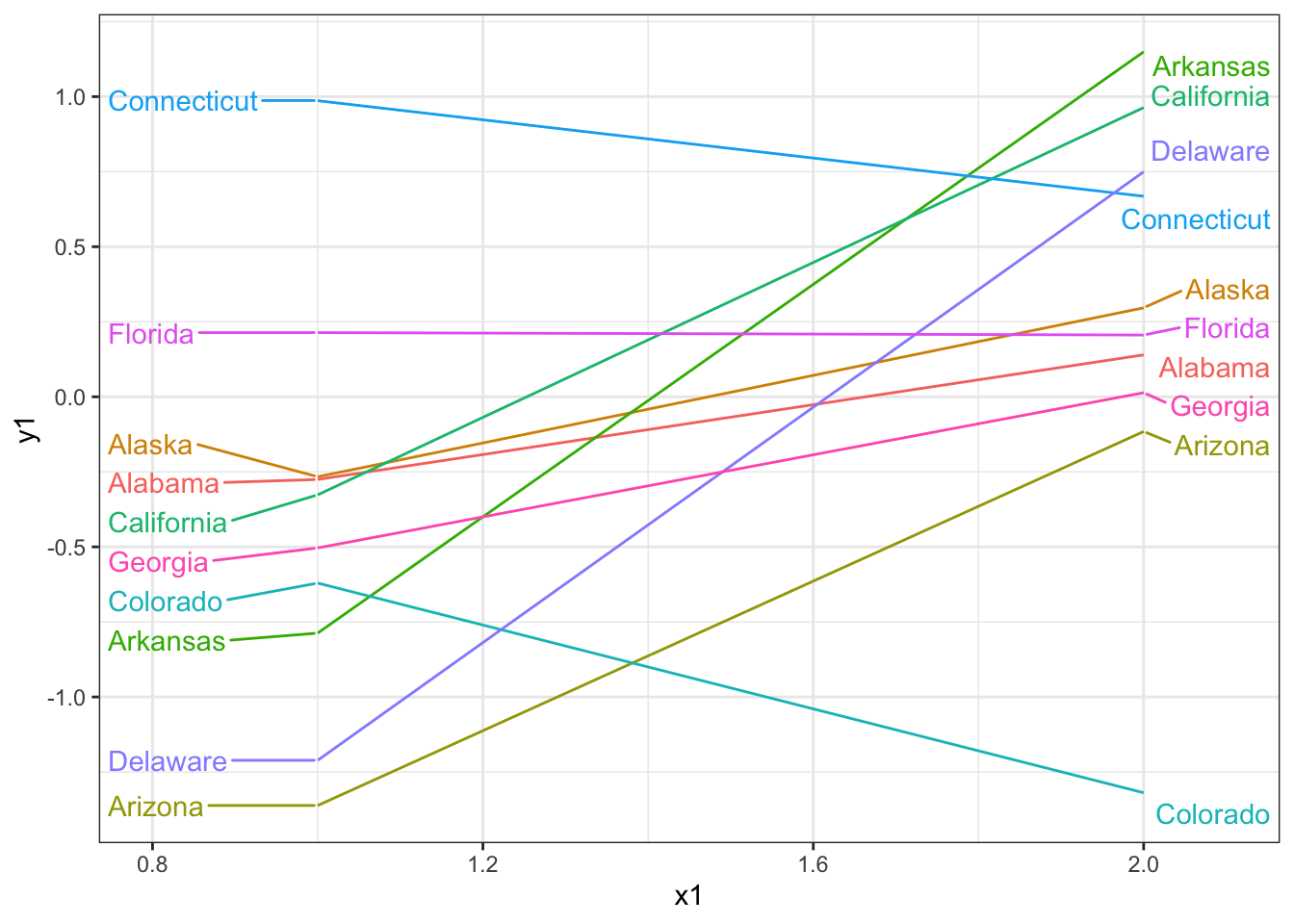## → Change label style properties

Thanks to the `color`, `bg.color` and `bg.r`, you can change colors of your labels.

Example:

``````mtcars\$car = rownames(mtcars)

ggplot(mtcars, aes(wt, mpg, label = car)) +
geom_point(color = "red") +
geom_text_repel(color = "white",     # text color
bg.color = "grey30", # shadow color